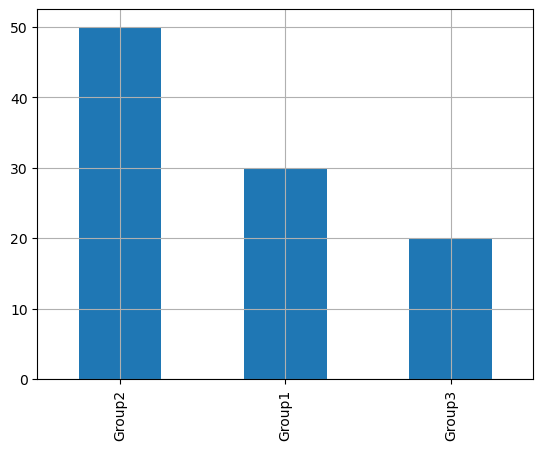A barplot is a graphical representation of data points in a dataset, where individual data points are represented by rectangular bars on a two-dimensional coordinate system. This type of plot allows us to visualize the distribution of categorical data by showing the frequency or count of each category along the plot.
Pandas, a powerful data manipulation library in Python, allows us to create bar plots with ease. In this post, we will explore how to leverage `Pandas` to create simple bar plots.

## Libraries

Pandas is a popular open-source Python library used for data manipulation and analysis. It provides data structures and functions that make working with structured data, such as tabular data (like `Excel` spreadsheets or `SQL` tables), easy and intuitive.

To install Pandas, you can use the following command in your command-line interface (such as `Terminal` or `Command Prompt`):

`pip install pandas`

Matplotlib functionalities have been integrated into the pandas library, facilitating their use with `dataframes` and `series`. For this reason, you might also need to import the matplotlib library when building charts with Pandas.

This also means that they use the same functions, and if you already know Matplotlib, you'll have no trouble learning plots with Pandas.

``````import pandas as pd
import matplotlib.pyplot as plt``````

## Dataset

In order to create graphics with Pandas, we need to use pandas objects: `Dataframes` and `Series`. A dataframe can be seen as an `Excel` table, and a series as a `column` in that table. This means that we must systematically convert our data into a format used by pandas.

Since barplots need qualitative variables, we will create a dataset with a categorical variable.

``````# Create a list with 3 different categories
category = ['Group1']*30 + ['Group2']*50 + ['Group3']*20

# Store the data into a dataframe
df = pd.DataFrame({'category': category})``````

## Barplot with the bar() function

Once we've opened our dataset, we'll now create the graph. An important feature of barplots in Python is that you can't simply give them a column name as an argument.

Instead, we have to calculate the counts for each category ourselves and give them as input to the function. To do this, we simply use the pandas `value_counts()` function.

``````# Get the repartition of each category
values = df['category'].value_counts()

# Create the plot
values.plot.bar(grid=True) # Add a grid in the background
plt.show()``````## Barplot with the plot() function

We'll now look at how to create a barplot using the `plot()` function. This function is very general and therefore requires more arguments to be specified when it is called.

The main argument is `kind`. This specifies the type of chart we want (in our case it's `'bar'`). For example, we could have put `'line'` for a line chart.

``````# Get the repartition of each category
values = df['category'].value_counts()

# Create the plot
values.plot(kind='bar', # type of chart
grid=True # Add a grid in the background
)
plt.show()``````## Going further

This post explains how to create a simple barplot with pandas in 2 different ways.

For more examples of how to create or customize your plots with Pandas, see the pandas section. You may also be interested in how to customize your barplots with Matplotlib and Seaborn.

## Contact & Edit

👋 This document is a work by Yan Holtz. You can contribute on github, send me a feedback on twitter or subscribe to the newsletter to know when new examples are published! 🔥